# CAT Prep 2022 | CAT Previous Year Question Papers With Solution | CAT 2022 Question Bank | PaGaLGuY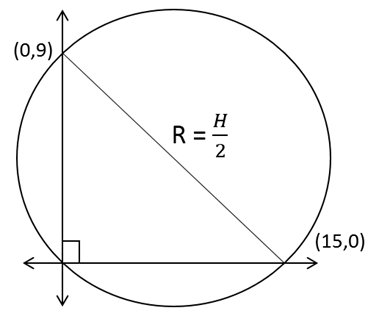We know that the equation of the straight line is 3x + 5y = 45The intercepts are (15,0) and (0,9) respectively
Since it’s a right-angled triangle, we know that Circumradius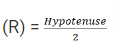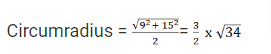We know thatis approximately equal to 6
So, from trial and error to find the closest number, we find that the value of Circumradius is very close to 9
So, the integer closest to L = 9

CAT 2019 Quant Question: Functions

For any positive integer n, let f(n) = n(n + 1) if n is even, and f(n) = n + 3 if n is odd. If m is a positive integer such that 8f(m + 1) - f(m) = 2, then m equals [TITA]

If n is even, f(n) = n (n + 1)
So, f (2) = 2(2+1) = 2 (3) = 6
If n is odd, f(n) = n + 3
f (1) = 1 + 3 = 4
It is given that, 8 x f(m+1) - f(m) =2
So, m can either be even or odd
Case-1: If m were even and m+1 odd
So, 8 x f(m+1) - f(m) =2
8(m + 4) - m (m + 1) = 2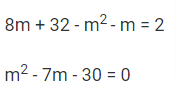(m-10) (m+3) = 0
m = 10 or -3
m = 10, since m is positive
Case-2: If m were even and m+1 odd
8 x f(m+1) - f(m) =2
8 (m +1) (m + 2) - (m + 3) = 2
Now, let us substitute m = 1 which is the minimum possible value
8 (1 + 1) (1 + 2) - (1 + 3)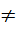3
Case 2 does not work